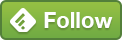## May 27 How To Find Outliers in Boxplots [via R Programming]

Finding outliers in Boxplots via Geom_Boxplot in R Studio

In the first boxplot that I created using GA data, it had ggplot2 + geom_boxplot to show google analytics data summarized by day of week.

While the min/max, median, 50% of values being within the boxes [inter quartile range] were easier to visualize/understand, these two dots stood out in the boxplot.

If the whiskers from the box edges describes the min/max values, what are these two dots doing in the geom_boxplot?

## Using a dplyr package to provide summary stats for geom_boxplot

After asking around, I found out a dplyr package that could provide summary stats for the boxplot [while I still haven't figured out how to add the data labels to the boxplot, the summary table seems like a good start].

This bit of the code creates a summary table that provides the min/max and inter-quartile range.

```gadata %>%
group_by(as.factor(dayofWeek)) %>%
summarise(Min = min(sessions),
Max = max(sessions),
Median = median(sessions),
IQRange = IQR(sessions))```

If you download the Xlsx dataset and then filter out the values where dayofWeek =0, we get the below values:

3, 5, 6, 10, 10, 10, 10, 11,12, 14, 14, 15, 16, 20

N = 14

Central values = 10, 11 [50% of values are above/below these numbers]

Median = (10+11)/2 or 10.5 [matches with the table above]

Smallest value = 3

Largest value = 20

Lower Quartile Value [Q1]: = (7+1)/2 = 4th value [below median range]= 10

Upper Quartile Value [Q3]: (7+1)/2 = 4th value [above median range] = 14

Inter Quartile Range = 14-10 = 4

If we want to know whether the first value  is an outlier here,

Lower outlier limit = Q1 - 1.5 * IQR = 10 - 1.5 *4

Lower outlier limit = 4

Upper outlier limit = Q3 + 1.5 *IQR = 14 + 1.5*4

Upper outlier limit = 20

As 3 is below the outlier limit, the min whisker starts at the next value ,

As all the max value is 20, the whisker reaches 20 and doesn't have any data value above this point.

And there's the geom_boxplot explained. Kinda cool it does all of this automatically!

Hope you found this blog post helpful

```#run the GA library
library(googleAnalyticsR)
#authentication token
ga_auth()

#setting up the query
gadata <- google_analytics(viewId = 55290473,
date_range = c(Sys.Date()-100, Sys.Date()-1),
metrics = c("users", "sessions", "pageviews"),
dimensions = c("date", "dayofWeek"),
anti_sample = TRUE)

#examining the first 6 rows as a preview
head(gadata)

#looking at the structure of the data
str(gadata)

#creating boxplot
boxplotchart <- ggplot(gadata, aes(x=dayofWeek, y = sessions)) +
geom_boxplot()

boxplotchart + ylab("Sessions on that day") + xlab("Day of Week; 0 = Sun, 6 = Sat")

boxplotchart <- ggplot(gadata,
aes(x = as.factor(dayofWeek),
y = sessions)) +
geom_boxplot()

boxplotchart

#table of boxplot data with summary stats
gadata %>%
group_by(as.factor(dayofWeek)) %>%
summarise(Min = min(sessions),
Max = max(sessions),
Median = median(sessions),
IQRange = IQR(sessions))

install.packages("xlsx")
library("xlsx")
write.xlsx(gadata, file = "C:\\Users\\KhanAd\\Dropbox\\blog content\\2018\\052018\\20180526 Day of week boxplot with outlier.xlsx",
sheetName = "Day Of Week", append = FALSE)
```